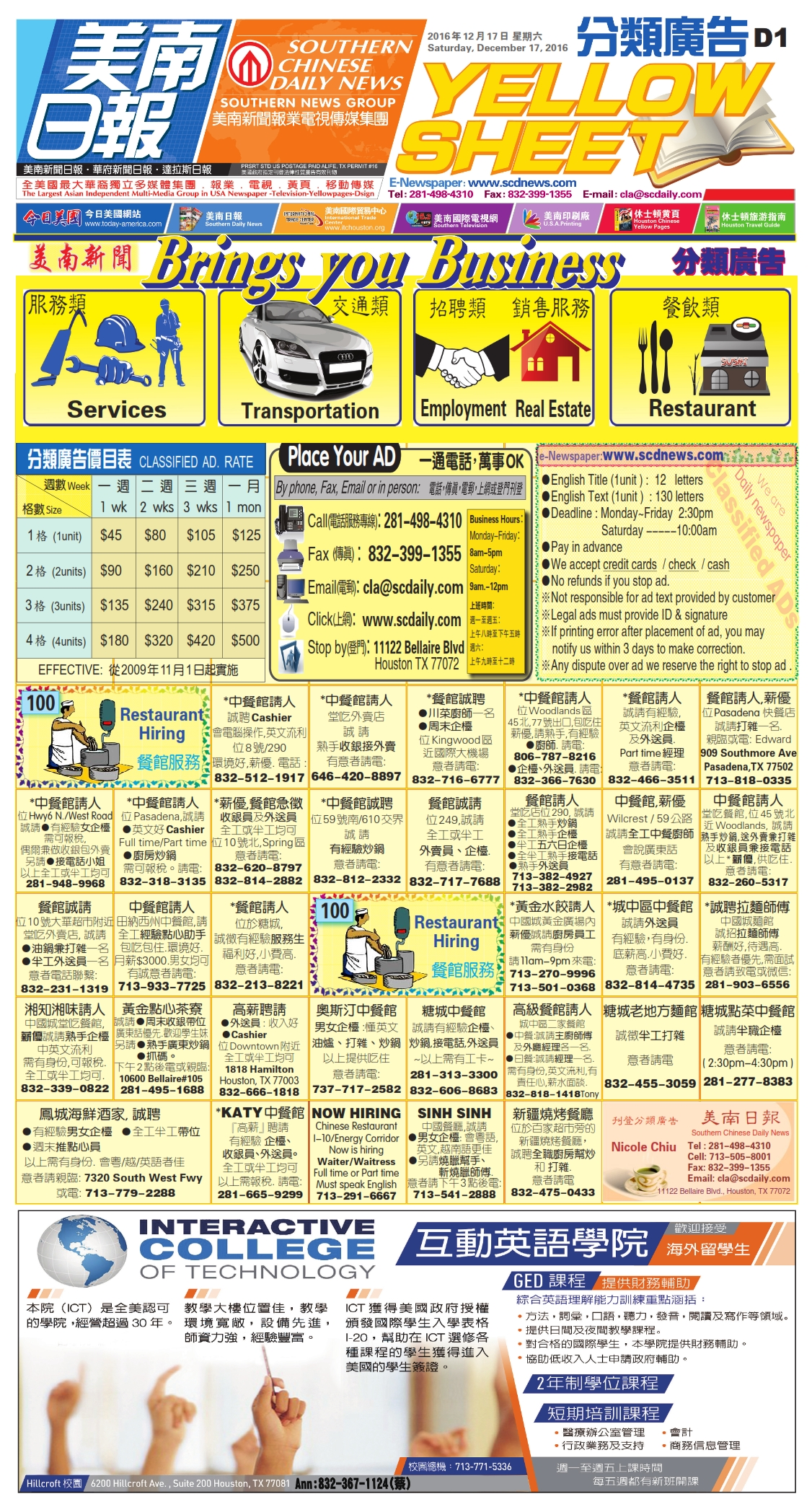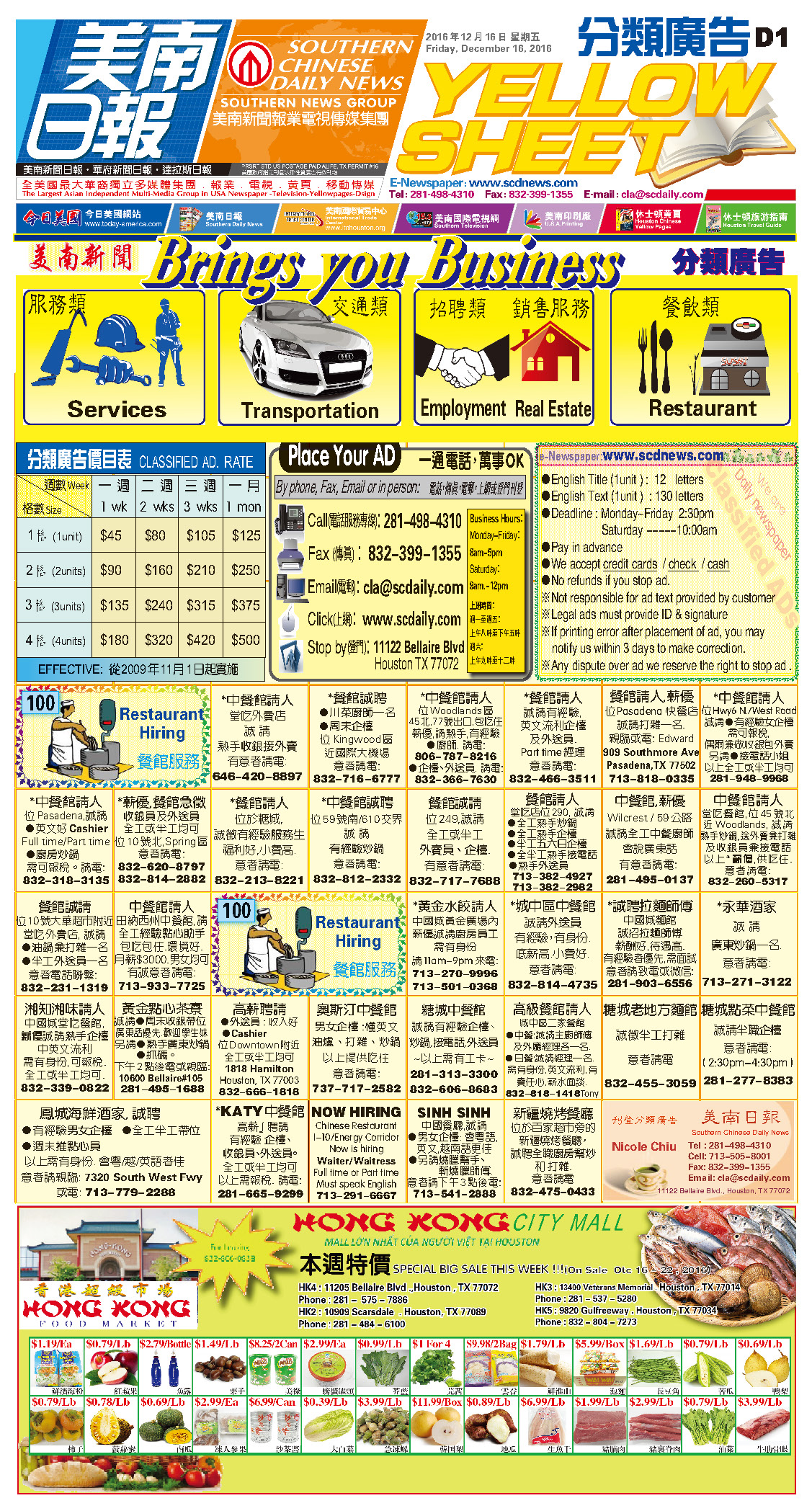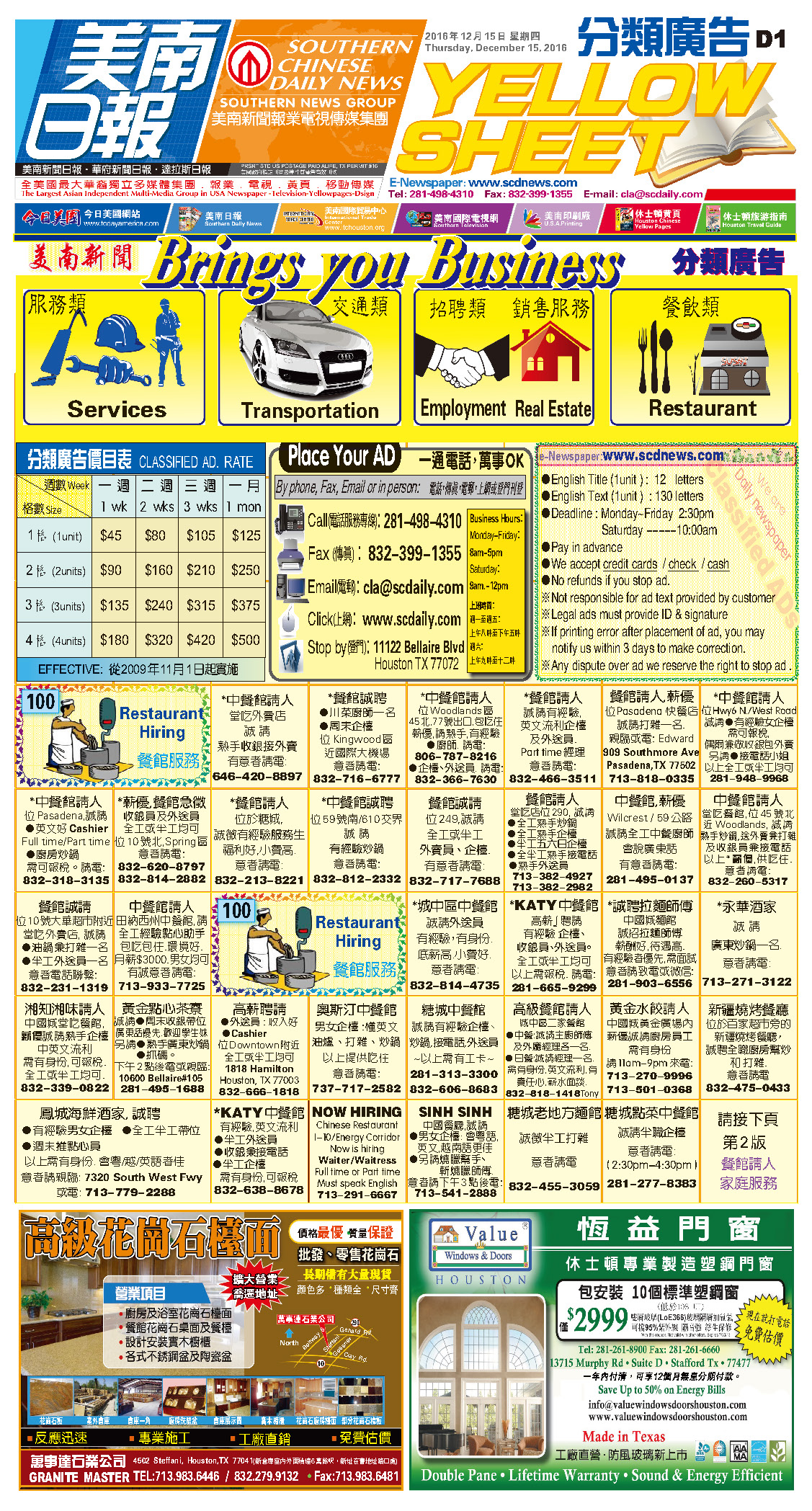161220 Epaper

 A Section B SectionC Section D Section161219 Epaper

 A Section B SectionC Section D Section161218 Epaper

 A Section B SectionC Section D Section161217 Epaper

 A Section B SectionC Section D Section161216 Epaper

 A Section B SectionC Section D Section161215 Epaper

 A Section B SectionC Section D Section161214 Epaper

 A Section B SectionC Section D Section161214 Epaper

 A Section B SectionC Section D Section161212 Epaper

 A Section B SectionC Section D Section161213 Epaper

 A Section B SectionC Section D Section161211 Epaper

 A Section B SectionC Section D Section